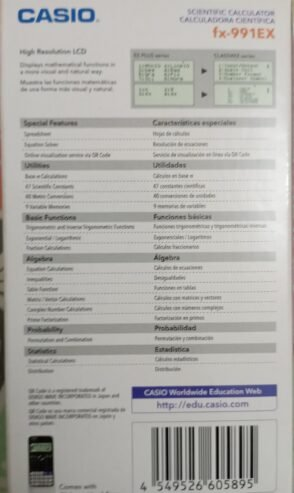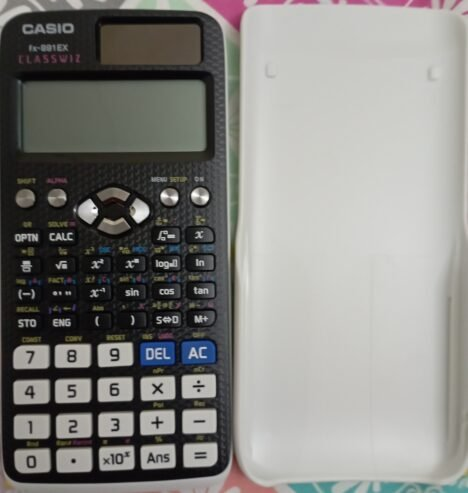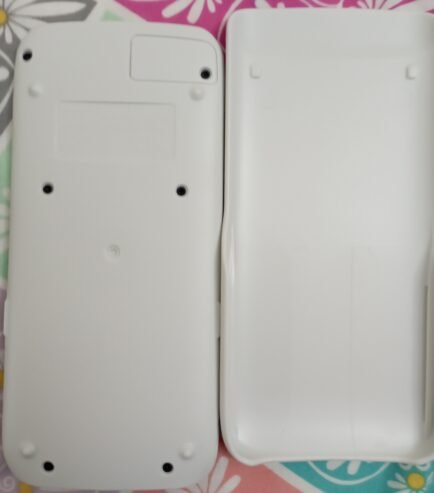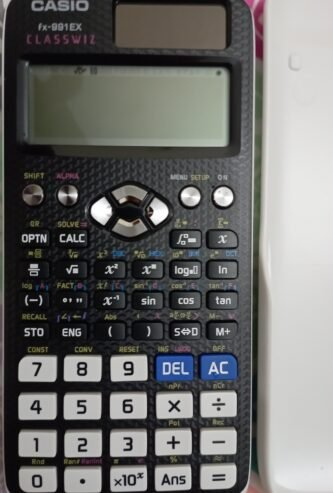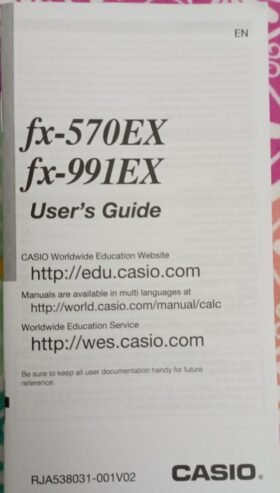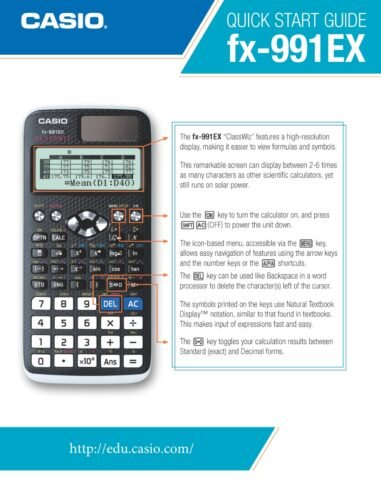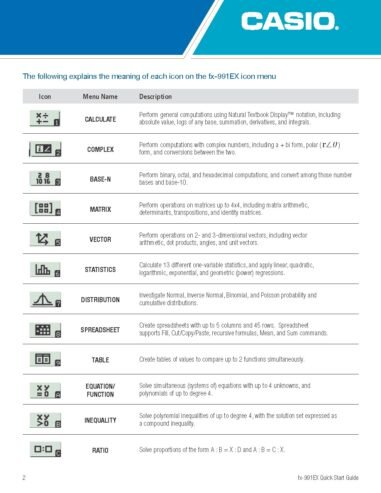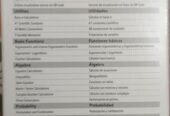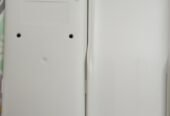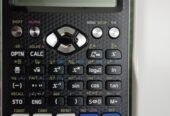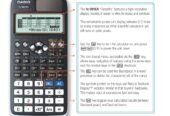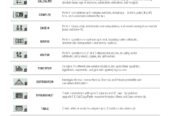Sold Out
• Sector 15-A/5, Buffer Zone, Gulberg, Karachi, Sindh, 75850, Pakistan
• Sell
FeaturedPopular
5,800

Original Casio Scientific Calculator, unused condition for sale in Karachi, Pakistan.

“CASIO SCIENTIFIC CALCULATOR fx-991EX for Education, Office and Home uses.”

CONDITION:

Original Genuine Product, customer can verify from calculator’s QR CODE upon inspection

Brand New

Unused condition

Excellent Condition

### Overview

• Category: Other Electronics
• Condition: New

### Features:

• CALCULATE Perform general computations using Natural Textbook Display™ notation, including absolute value, logs of any base, summation, derivatives, and integrals.
• COMPLEX Perform computations with complex numbers, including a + bi form, polar ( ) form, and conversions between the two.
• BASE-N Perform binary, octal, and hexadecimal computations, and convert among those number bases and base-10.
• MATRIX Perform operations on matrices up to 4x4, including matrix arithmetic, determinants, transpositions, and identity matrices.
• VECTOR Perform operations on 2- and 3-dimensional vectors, including vector arithmetic, dot products, angles, and unit vectors.
• STATISTICS Calculate 13 different one-variable statistics, and apply linear, quadratic, logarithmic, exponential, and geometric (power) regressions.
• DISTRIBUTION Investigate Normal, Inverse Normal, Binomial, and Poisson probability and cumulative distributions.
• SPREADSHEET Create spreadsheets with up to 5 columns and 45 rows. Spreadsheet supports Fill, Cut/Copy/Paste, recursive formulas, Mean, and Sum commands.
• TABLE Create tables of values to compare up to 2 functions simultaneously.
• EQUATION/FUNCTION
• Solve simultaneous (systems of) equations with up to 4 unknowns, and polynomials of up to degree 4.
• INEQUALITY Solve polynomial inequalities of up to degree 4, with the solution set expressed as a compound inequality.
• RATIO Solve proportions of the form A : B = X : D and A : B = C : X.

### Location

Sector 15-A/5, Buffer Zone, Gulberg, Karachi, Sindh, 75850, Pakistan